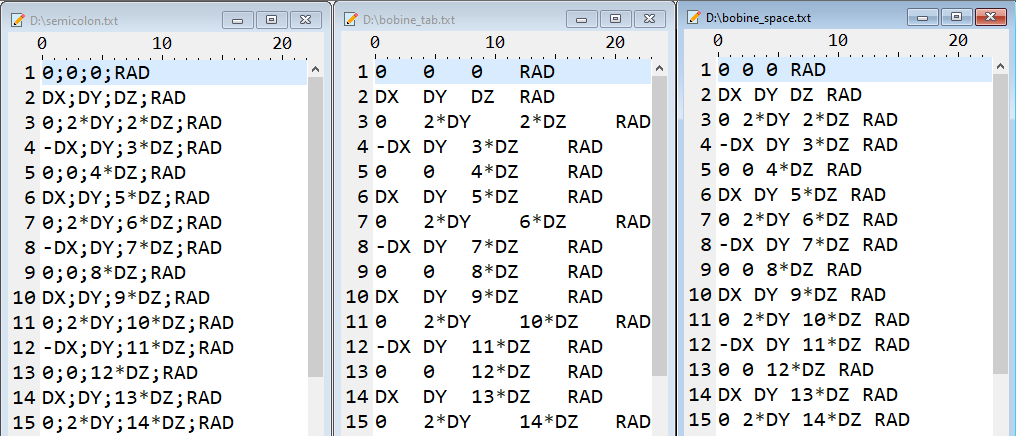# Composed coil

## Shape and dimension

Composed coils are ones which complex shape is defined by the user.

The shape of the coil is the path (open or closed) composed by a set of points. The connecting elements between the points are built on the basis of straight segments with taking into account of the curves if they exist, as presented on the figure below. A curvature radius, associated to the point, defines the curve.

Composed coil with closed path, described by 3 points

Composed coil with open path, described by 3 pointsa curvature radius R = 0

a curvature radius R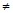0 *

is associated to the point P 2

Note: * The value of a curvature radius R in a specified point must be between 0 and the minimum of the distances (the distance between this point and the point which precedes it and the distance between this point and the point which follows it).

## Current direction

A coil is defined in a proper coordinate system. The direction of the current is dependent on the orientation of this coordinate system and the arrow on the image of the coil defines the direction of the positive current in the coil.

In the composed coil the direction of the current follows the succession of the points. The user can easily change the current direction by reversing the order of the points via the button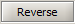.

## Sections

The types of associated sections are presented in the table below.

Section Composed coil

Line *Disc

(volume displaying)Disc

(the mean fiber displaying)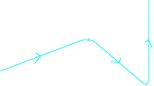RectangleNote: * The thickness value must be one order of magnitude lower than the height of the current sheet (for example, hundred times lower).

## Orientation of a rectangular section

For rectangular sections (sections of line or rectangle type), it is necessary to define the position of section as presented in the figure below.

The orientation of section is defined in the plane perpendicular to mean fiberThere are two different situations:

• if a coil is formed of several not aligned segments :

the orientation is predefined and fixed by Flux

• if a coil is formed of only one segment (or several aligned segments ):

the orientation is free and defined by the user

These two situations are detailed in following blocks.

## Orientation (1)

The orientation for a coil formed of several not aligned segments is imposed by Flux. The orientation of a section is a function of the coil shape, i.e. of its segments positioning.

The user can define a rotation angle of 90° to make turn the rectangular section of 90°; but that is equivalent to swap the height and width values.

In the example below the section of rectangle type is oriented using the rotation angle.

Rotation angle = 0° Rotation angle = 90°## Orientation (2)

The orientation for a coil formed of only one segment (or of some aligned segments ) is defined using an orientation vector and a rotation angle (optional) as represented in the table below.

• an orientation vector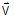gives the direction of the width / thickness of the section for an angle of 0° in the plane of the section (see figure below)• a rotation angle α (optional) is the angle of the clockwise section rotation in the plane of the section (see figure below)## Example of orientation

In the example below the section is oriented using:

• the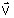vector to define the width (or the thickness) position
• the rotation angle equal to 0°Note:

If the vectoris not in the plane of the section, Flux projects it in this plane.

The vectorshould not be collinear with the segments.

## Help for creation

To facilitate the creation of composed coils (non meshed), several features are available in the creation dialog box :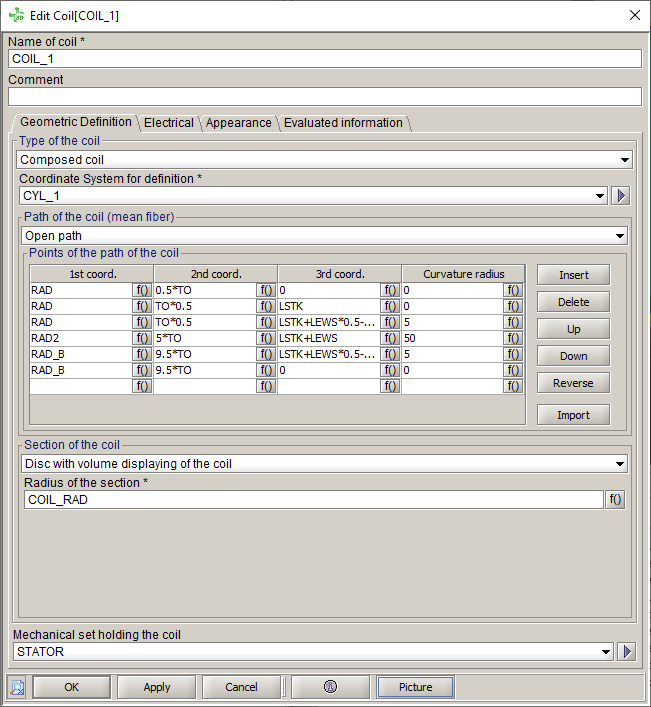• Insert a point in the list via the button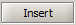.
• Delete a point in the list via the button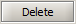(after activating one of the cells of the line to be deleted).

The definition line of the point is fully deleted, i.e. the 3 coordinates as well as the curvature radius.

• Move a point up or down using the buttons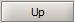and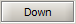(after activating one of the cells of the line to be deleted).
• Reverse the order of the points in the table using the button(allows, among other things, to easily change the direction of the current in the coil).
• Import a list of points contained in a TXT file in order to fill the points table with the desired list of points via the button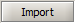.

If the table already contains points, the import will overwrite them all and replace them with the list of points of the imported file.

The file must contain 4 columns: the coordinates X, Y, Z and the value of the curvature radius. The separator can be:

• a semicolon
• a space
• a tabulation